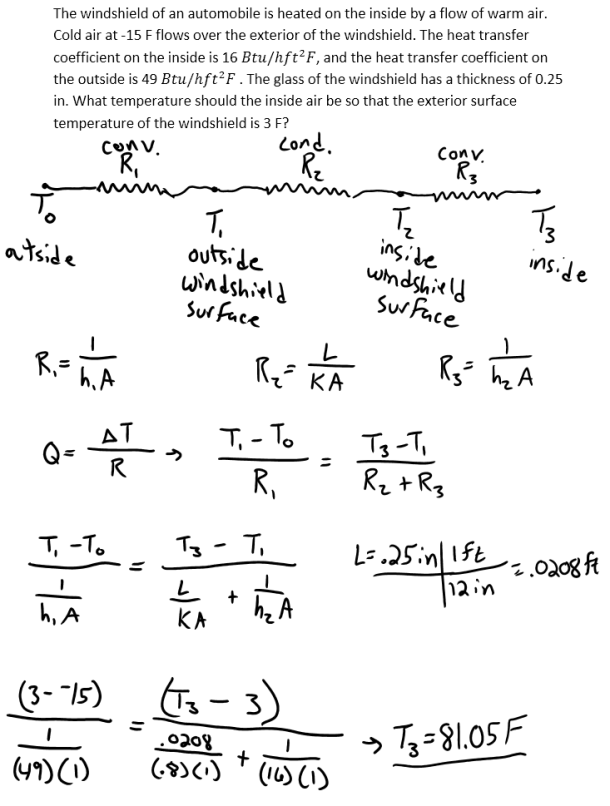heat and mass transfer problems engineering equations heat and mass transfer school homework engineering heat and mass transfer formulas heat and mass transfer solutions to heat and mass transfer problems full solution engineering problem solution heat and mass transfer math problems engineering equations heat and mass transfer school homework engineering solutions to heat and mass transfer formulas heat problems mass problem solutions to transfer problems full solution heat and mass transfer
heat and mass transfer problems engineering equations heat and mass transfer school homework engineering heat and mass transfer formulas heat and mass transfer solutions to heat and mass transfer problems full solution engineering problem solution heat and mass transfer math problems engineering equations heat and mass transfer school homework engineering solutions to heat and mass transfer formulas heat problems mass problem solutions to transfer problems full solution heat and mass transfer
Highalphabet Home Page heat and mass transfer problem solutions Heat and Mass Transfer Page
The windshield of an automobile is heated on the inside by a flow of warm air. Cold air at -15 F flows over the exterior of the windshield. The heat transfer coefficient on the inside is 16 Btu/hft^2 F, and the heat transfer coefficient on the outside is 49 Btu/hft^2 F . The glass of the windshield has a thickness of 0.25 in. What temperature should the inside air be so that the exterior surface temperature of the windshield is 3 F?The windshield of an automobile is heated on the inside by a flow of warm air. Cold air at -15 F flows over the exterior of the windshield. The heat transfer coefficient on the inside is 16 Btu/hft^2 F, and the heat transfer coefficient on the outside is 49 Btu/hft^2 F . The glass of the windshield has a thickness of 0.25 in. What temperature should the inside air be so that the exterior surface temperature of the windshield is 3 F?